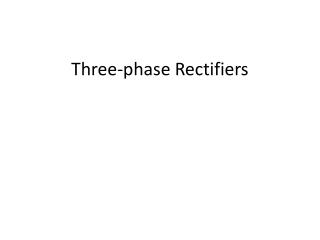DownloadDownload PresentationThree-phase Rectifiers

# Three-phase Rectifiers

Download Presentation## Three-phase Rectifiers

- - - - - - - - - - - - - - - - - - - - - - - - - - - E N D - - - - - - - - - - - - - - - - - - - - - - - - - - -
##### Presentation Transcript

1. Three-phase Rectifiers

2. Rectifiers (SEE 4433) Half wave Full wave Single-phase Three-phase Single-phase Uncontrolled (diode) Controlled (SCR) Uncontrolled (diode) Uncontrolled (diode) Controlled (SCR) Controlled (SCR)

3. Uncontrolled (diode) Three-phase Rectifiers • At any one time, only ONE of the upper diodes can conduct • The most forward biased diode will conduct – this will depend on the instantaneous phase voltage since the upper diodes have a common cathode • At any one time, only ONE of the lower diodes can conduct • The most forward biased diode will conduct – this will depend on the instantaneous phase voltage since the lower diodes have a common anode

4. Uncontrolled (diode) Three-phase Rectifiers • At any one time, only ONE of the upper diodes can conduct • The most forward biased diode will conduct – this will depend on the instantaneous phase voltage since the upper diodes have a common cathode • At any one time, only ONE of the lower diodes can conduct • The most forward biased diode will conduct – this will depend on the instantaneous phase voltage since the lower diodes have a common anode

5. Uncontrolled (diode) Three-phase Rectifiers • The load voltage, vo, is the difference between the phase voltage (line-line voltage) For example, when D1 and D6 conduct, using KVL -van +vO +vbn = 0 ==> vO = van – vbn = vab

6. Uncontrolled (diode) Three-phase Rectifiers • The load voltage, vo, is the difference between the phase voltage (line-line voltage) When D1 and D2 conduct, using KVL -van +vO +vcn = 0 ==> vO = van – vcn = vac

7. Uncontrolled (diode) Three-phase Rectifiers

8. Uncontrolled (diode) Three-phase Rectifiers

9. Controlled (SCR) Three-phase Rectifiers • The transition of output voltage to the maximum instantaneous line-line voltage can be delayed • The reference of delay angle is when the SCR should conduct if it is a diode

10. Controlled (SCR) Three-phase Rectifiers

11. Controlled (SCR) Three-phase Rectifiers Delay angle beyond 90o is possible if the load is active (e.g. DC motor). The power is transferred from the load to the source.